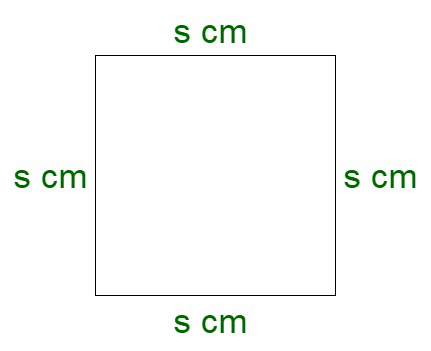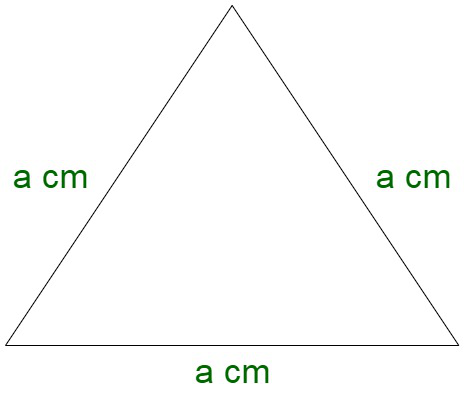# One side of an equilateral triangle is 8 cm, what is the area of the square of the perimeter be same.

• Last Updated : 10 Nov, 2021

Square is a geometrical figure which is formed of four equal sides. It is a regular quadrilateral where all the angles are right angles. Basically, it can be considered as a special case of a rectangle, where all the sides are equal in length. For instance, a square courtyard.Properties of square

• All four sides of a square are equal.
• The diagonals of a square are equal.
• All the four angles of a square are equal.
• The diagonals of a square bisect its angles.

### Perimeter of a square

The perimeter of a square is referred as the total length of the boundary enclosing the geometrical figure.

Perimeter of square = Sum of all sides of a square

Let us assume s to be the side of the square.

Since, we know, all the sides of the square are equal.

Perimeter of square = s + s + s + s

Perimeter of square = 4s ….. (I)

### Area of a square

Therefore, area of the square is given by,

Area of square = s2 ………(II)

### Equilateral triangleAn equilateral triangle is a geometrical 2D figure which has all three sides equal. All the edges subtend an angle of 60o at the corners. Therefore, the sum of all the angles of the triangle is 180°.

Properties of an equilateral triangle

• All three sides of an equilateral triangle are equal.
• All the three angles are equal i.e 60° each.
• A regular polygon has three equal sides.

### Perimeter of an equilateral triangle

The perimeter of a square is referred to as the total length of the boundary enclosing the geometrical figure.

Perimeter of equilateral triangle = Sum of all sides of an equilateral triangle

Let us assume a to be the side of an equilateral triangle.

Since, we know, all the sides of the equilateral triangle are equal.

Perimeter of equilateral triangle = a + a + a

Perimeter of equilateral triangle = 3a……. (III)

### One side of an equilateral triangle is 8 cm, what is the area of the square if the perimeter be the same.

Solution:

Since, we know,

Perimeter of square = 4s  …(By I)

Perimeter of equilateral triangle = 3a … (By III)

As per the question, we get, equating we get,

Perimeter of square = Perimeter of equilateral triangle

4s = 3a

On solving, we get,

=> s = 3/4 a

Now, we have, side of equilateral triangle = 8 cm

s = (3/4) * 8 cm

s = 6 cm

Therefore, the side of the square = 6 cm

Now,

Area of a square = s2 …(By II)

On substituting the values, we get,

Area of a square = 62

= 36 sq. cm.

Therefore, the area of a square = 36 sq. cm.

### Sample Questions

Question 1: Assume that the perimeter of the square and triangle are the same. If one side of an equilateral triangle is 16 cm. Then find the perimeter of an equilateral triangle and the square?

Solution:

Here,

We have one side of the equilateral triangle 16 cm

we are given that the perimeter of the equilateral triangle and square are the same

Perimeter of Equilateral triangle = Side + Side + Side = 3a

Perimeter of Square = Side + Side + Side + Side = 4s

As per the question

Perimeter of Equilateral triangle = Perimeter of Square

3a = 4s

We have a = 16 cm

3 × 16 = 4 × s

48 = 4 × s

s =s = 12 cm

Therefore,

Perimeter of Equilateral triangle = 3a

= 3 × 16

= 48 cm

Perimeter of Square = 4s

= 4 × 12

= 48 cm

Question 2: Assume that the perimeter of the square and triangle are the same. If one side of the equilateral triangle is 12 cm. Then find the area of the square?

Solution:

Here,

We have one side of the equilateral triangle 12 cm

we are given that the perimeter of the equilateral triangle and square are the same

Perimeter of Equilateral triangle = Side + Side + Side = 3a

Perimeter of Square = Side + Side + Side + Side = 4s

As per the question

Perimeter of Equilateral triangle = Perimeter of Square

3a = 4s

We have a = 12 cm

3 × 12 = 4 × s

36 = 4 × s

s =s = 9 cm

Side of square is 9 cm.

Now we have to find the area of square

Area of Square = Side × Side

= 9 × 9

= 81 cm2

Area of Square = 81 cm2

Question 3: Consider that the perimeter of an Equilateral triangle and a square are the same and one side of the square is 15 cm. Then Find the perimeter of the square and sides of the Equilateral triangle.

Solution:

Here,

We are given that one side of square = 15 cm

Perimeter of Square = 4 × Side

= 4 × 15

= 60 cm

Perimeter of Equilateral triangle = 3 × side

As per the question perimeter of the Equilateral triangle is equal to the perimeter of the square

Therefore,

Perimeter of Equilateral triangle = 3 × side = 60 cm

60 cm = 3 × Side

Side =Side of Equilateral triangle = 20 cm

Question 4: Consider that the perimeter of an Equilateral triangle and a Square are the same. Then Find the ratio of their areas?

Solution:

Here,

We have to find the ratio of the area of equilateral triangle and area of square

Assume the side of the triangle be a

Thus the perimeter of triangle = 3a

Assume the side of square be s

Thus the perimeter of square = 4s

As per the question

Perimeter of Equilateral Triangle = Perimeter of Square

3a = 4s

a =Then,

Area of Equilateral triangle =Further

Area of square = s2

Now find the ratio of their areasQuestion 5: Assume that the perimeter of the square and triangle are the same. If one side of an equilateral triangle is 32 cm. Then find the perimeter of an equilateral triangle and the square?

Solution:

Here,

We have one side of equilateral triangle 32 cm

we are given that the perimeter of the equilateral triangle and square are the same

Perimeter of Equilateral triangle = Side + Side + Side = 3a

Perimeter of Square = Side + Side + Side + Side = 4s

As per the question

Perimeter of Equilateral triangle = Perimeter of Square

3a = 4s

We have a = 32 cm

3 × 32 = 4 × s

96 = 4 × s

s =s = 24 cm

Therefore,

Perimeter of Equilateral triangle = 3a

= 3 × 32

= 96 cm

Perimeter of Square = 4s

= 4 × 24

= 96 cm

My Personal Notes arrow_drop_up